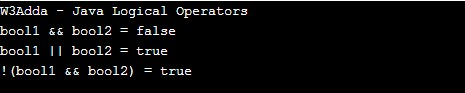# Java Logical Operators

In this tutorial you will learn about the Java Logical Operators and its application with practical example.

## Java Logical Operators

Logical operators are used to combine expressions with conditional statements using logical (AND,OR,NOT) operators, which results in true or false. Let variable a holds true or 1 and variable b holds false or 0, then −

Java Logical operators
Operator Name Description Example
`&&` `Logical AND` `return true if all expression are true` ```(a && b) returns false ```
`||` `Logical OR` `return true if any expression is true` ```(a || b) returns true ```
`!` `Logical NOT` `return complement of expression` `!a returns false`

Example:-

When you run the above java program, you will see the following output.

Output:-In this tutorial we have learn about the Java Logical Operators and its application with practical example. I hope you will like this tutorial.